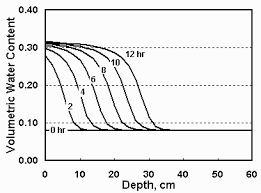## How to Calculate and Solve for Quantity of Soil Moved | Water BudgetThe image above represents quantity of soil moved.

To compute for quantity of soil moved, two essential parameters are needed and these parameters are Excess Wind Velocity (vw) and Particle Diameter (dp).

The formula for calculating quantity of soil moved:

Q = vw³ . dp

Where;

Q = Quantity of Soil Moved
vw = Excess Wind Velocity
dp = Particle Diameter

Let’s solve an example;
Find the quantity of soil moved when the excess wind velocity is 12 and the particle diameter is 20.

This implies that;

vw = Excess Wind Velocity = 12
dp = Particle Diameter = 20

Q = vw³ . dp
Q = (12)³ . (20)
Q = (1728) . (20)
Q = 34560

Therefore, the quantity of soil moved is 34560.

Calculating the Excess Wind Velocity when the Quantity of Soil Moved and the Particle Diameter is Given.

vw³ = 3Q / dp

Where;

vw = Excess Wind Velocity
Q = Quantity of Soil Moved
dp = Particle Diameter

Let’s solve an example;
Find the excess wind velocity when the quantity of soil moved is 30 and the particle diameter is 10.

This implies that;

Q = Quantity of Soil Moved = 30
dp = Particle Diameter = 10

vw³ = 3Q / dp
vw³ = 330 / 10
vw³ = 3√3
vw³ = 1.442

Therefore, the excess wind velocity is 1.442.